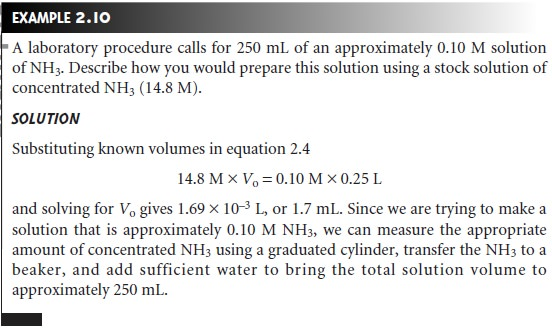Home | | Modern Analytical Chemistry | Preparing Solutions of Analytical Chemistry

# Preparing Solutions of Analytical Chemistry

1. Preparing Stock Solutions 2. Preparing Solutions by Dilution

Preparing Solutions

Preparing a solution of known concentration is perhaps the most common activity in any analytical lab. The method for measuring out the solute and solvent depend on the desired concentration units, and how exact the solution’s concentration needs to be known. Pipets and volumetric flasks are used when a solution’s concen- tration must be exact; graduated cylinders, beakers, and reagent bottles suffice when concentrations need only be approximate. Two methods for preparing solutions are described in this section.

## Preparing Stock Solutions

A stock solution is prepared by weighing out an appropriate portion of a pure solid or by measuring out an appropriate volume of a pure liquid and diluting to a known volume. Exactly how this is done depends on the required concentration units. For example, to prepare a solution with a desired molarity you would weigh out an appropriate mass of the reagent, dissolve it in a portion of solvent, and bring to the desired volume. To prepare a solution where the solute’s concentration is given as a volume percent, you would measure out an appropriate volume of solute and add sufficient solvent to obtain the desired total volume.## Preparing Solutions by Dilution

Solutions with small concentrations are often prepared by diluting a more concen- trated stock solution. A known volume of the stock solution is transferred to a new container and brought to a new volume. Since the total amount of solute is the same before and after dilution, we know that

Co x Vo = Cd x Vd              ………………….2.4

where Co is the concentration of the stock solution, Vo is the volume of the stock solution being diluted, Cd is the concentration of the dilute solution, and Vd is the volume of the dilute solution. Again, the type of glassware used to measure Vo and Vd depends on how exact the solution’s concentration must be known.As shown in the following example, equation 2.4 also can be used to calculate a solution’s original concentration using its known concentration after dilution.Study Material, Lecturing Notes, Assignment, Reference, Wiki description explanation, brief detail
Modern Analytical Chemistry: Basic Tools of Analytical Chemistry : Preparing Solutions of Analytical Chemistry |